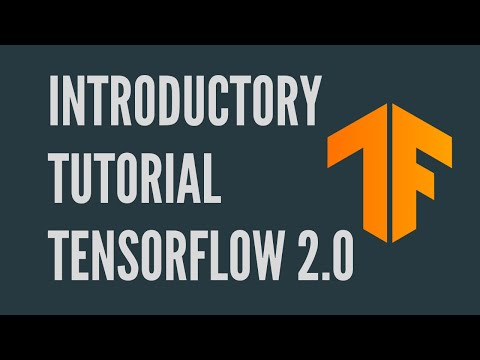TensorFlow 2.0 - Introductory Tutorial

TensorFlow 2.0 is here! Let’s take a look at a simple tutorial on the basics of TensorFlow.

To view the video